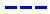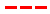Magic Kingdom attraction wait times forecast for December 25, 2015.

•The Average Wait Time We PredictedThe Average Wait Time We Saw
•The Average Wait Time We PredictedThe Average Wait Time We Saw
•The Average Wait Time We PredictedThe Average Wait Time We Saw
•The Average Wait Time We PredictedThe Average Wait Time We Saw
•The Average Wait Time We PredictedThe Average Wait Time We Saw
•The Average Wait Time We PredictedThe Average Wait Time We Saw
•The Average Wait Time We PredictedThe Average Wait Time We Saw
•The Average Wait Time We PredictedThe Average Wait Time We Saw
•The Average Wait Time We PredictedThe Average Wait Time We Saw
•The Average Wait Time We PredictedThe Average Wait Time We Saw
•The Average Wait Time We PredictedThe Average Wait Time We Saw
•The Average Wait Time We PredictedThe Average Wait Time We Saw
•The Average Wait Time We PredictedThe Average Wait Time We Saw
•The Average Wait Time We PredictedThe Average Wait Time We Saw
•The Average Wait Time We PredictedThe Average Wait Time We Saw
•The Average Wait Time We PredictedThe Average Wait Time We Saw
•The Average Wait Time We PredictedThe Average Wait Time We Saw
•The Average Wait Time We PredictedThe Average Wait Time We Saw
•The Average Wait Time We PredictedThe Average Wait Time We Saw
•The Average Wait Time We PredictedThe Average Wait Time We Saw
•The Average Wait Time We PredictedThe Average Wait Time We Saw
•The Average Wait Time We PredictedThe Average Wait Time We Saw
•The Average Wait Time We PredictedThe Average Wait Time We Saw
•The Average Wait Time We PredictedThe Average Wait Time We Saw
•The Average Wait Time We PredictedThe Average Wait Time We Saw
•The Average Wait Time We PredictedThe Average Wait Time We Saw
•The Average Wait Time We PredictedThe Average Wait Time We Saw
•The Average Wait Time We PredictedThe Average Wait Time We Saw
•The Average Wait Time We PredictedThe Average Wait Time We Saw
•The Average Wait Time We PredictedThe Average Wait Time We Saw
•The Average Wait Time We PredictedThe Average Wait Time We Saw
•The Average Wait Time We PredictedThe Average Wait Time We Saw